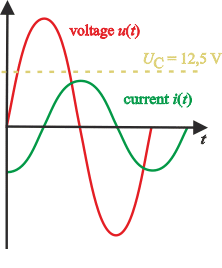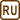## Instantaneous Voltage on Generator

A generator with an instantaneous voltage u(t) = 25sin(377t) is connected to a coil of inductance 12.7 H.

a) What is the maximum value of the electric current?
b) What is the generator voltage value when the current is of maximum value?
c) What is the current value when the voltage is 12.5 V and decreases?

Note: We do not add units to the equation for instantaneous voltage and current. We do not accept multiplied units, i.e. we substitute time in seconds and so on.

• #### Given values

We know from the assignment:

 Instantaneous generator voltage: u(t) = 25sin(377t) Inductance of coil: L = 12.7 H

We want to determine:

 a) Current amplitude: Im = ? (A) b) Voltage, if current is at maximum: Ub = ? (V) c) Current, if voltage is Uc = 12.5 V: Ic = ? (A)

• #### Hint 1

What do the quantities in the formula for instantaneous voltage mean?

• #### Hint 2

To calculate the maximum current value or the amplitude of current, we use Ohm's law for alternating current.

• #### Hint 3

What is the phase difference between the voltage and current if there is a coil in the circuit?

• #### Analysis

The task is divided into three parts, which will be solved successively.

a) If we know the behaviour of the instantaneous voltage in the circuit, we know the voltage amplitude. The current amplitude (= maximum current) is obtained from Ohm's law. The impedance in Ohm's law comprises only from the impedance of the coil, which can be calculated from the coil inductance.

b) First, we express the behaviour of instantaneous current in the circuit. A coil is connected in this circuit, i.e. the voltage leads the current by π/2. Then we determine the time at which the current reaches its maximum value. We insert this time into the voltage waveform.

c) The procedure in this part will be similar to the one in part b). We evaluate time t, in which the voltage is 12.5 V. Because the behaviour is described by a sine function, we obtain more time values corresponding to this voltage. We choose only such values, for which the voltage decreases and we insert them in the equation for the instantaneous value of the current.

• #### Analysis of formula describing instantaneous voltage

We compare the general formula for instantaneous voltage with the given dependency:

$u(t)=U_m\sin\left(\omega t+ {\varphi}_{0u}\right)=25\sin\left(377t\right)$

From there we see that:

$U_m=25\,\mathrm V$ $\omega=377\,\mathrm s^{-1}$
• #### a) Solution

We apply Ohm's law

$I_m=\frac{U_m}{Z}$

and we substitute the inductance of the coil for the impedance Z:

$I_m=\frac{U_m}{Z}=\frac{U_m}{X_L}=\frac{U_m}{\omega L}$

We insert the given values:

$I_m=\frac{25}{377 {\cdot} 12{.}7}\,\mathrm A\,\dot{=}\,5{.}2 \,\mathrm mA$

• #### b) Solution

We write the equation expressing the current instantaneous value. In section a) we have determined the current amplitude; the phase difference between the current and voltage is -π/2, because there is only a coil in the circuit. The voltage leads the current.

$i\left(t\right)=I_m \sin\left(\omega t + {\varphi}_{0i}\right)=5.2 {\cdot} 10^{-3}\sin\left(377t- \frac{\pi}{2}\right)$

We want to find the moment when the current is maximum, it means that i(t) = Im:

$i\left(t\right)=I_m\,\sin\left(377t-\frac{\pi}{2}\right)$ $I_m=I_m\,\sin\left(377t-\frac{\pi}{2}\right)$ $1=\sin\left(377t-\frac{\pi}{2}\right)$ $\frac{\pi}{2}=377t-\frac{\pi}{2}$ $t=\frac{\pi}{377}\,\mathrm s$

The voltage at the maximum current is obtained by substituting into the formula, which expresses the instantaneous voltage value::

$U_b=25\,\sin\left(377t\right)=25\,\sin\left(377 \cdot \frac{\pi}{377}\right)\,\mathrm V=25\,\sin\left(\pi\right)\,\mathrm V=0\,\mathrm V$

We could have guessed the zero voltage value at maximum current even without calculation, since both waveforms of instantaneous values of current and voltage are described by a sine function. In a case of the coil, the phase difference of the sine functions is exactly π/2. So, if one of the sine functions is at a maximum value, the other must be at minimum. Look at the picture in the next section.

• #### c) Solution

We want to determine the magnitude of the current if the voltage is Uc = 12.5 V.

$U_c=25\sin\left(377t\right)$ $12{,}5=25\,\sin\left(377t\right)$ $\sin\left(377t\right)=\frac{1}{2}$

We solve this task only for the first cycle of the sine function describing the behaviour of the instantaneous voltage. We would obtain the same results in following cycles. We get two time values at which the voltage reaches the requested value:

$377t= \frac{\pi}{6 }\,\mathrm s \tag{1}$ $377t= \frac{5\pi}{6 }\,\mathrm s \tag{2}$

To choose the right value, we plot the waveform of voltage and current in a diagram.In the figure the yellow dotted line represents the voltage of 12.5 V.

The value we need is: $$377t= \frac{5\pi}{6 }\,\mathrm s$$, because the voltage decreases

We substitute this value into the formula for calculating the instantaneous current value and we obtain the value of current if the voltage is 12.5 V:

$I_c=5.2 {\cdot} 10^{-3}\sin\left(377t- \frac{\pi}{2}\right)=5.2 {\cdot} 10^{-3}\sin\left( \frac{5\pi}{6 } - \frac{\pi}{2}\right)\,\mathrm A=$ $\ =5.2 {\cdot} 10^{-3}\sin\left(\frac{\pi}{3}\right)\,\mathrm A\,\dot{=}\,4{.}5\,\mathrm mA$